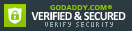ISBN: 1470437724

ISBN13: 9781470437725

# Automorphisms of Fusion Systems of Finite Simple Groups of Lie Type (Memoirs of the American Mathematical Society)

For a finite group $G$ of Lie type and a prime $p$, the authors compare the automorphism groups of the fusion and linking systems of $G$ at $p$ with the automorphism group of $G$ itself. When $p$ is the defining characteristic of $G$, they are all isomorphic, with a very short list of exceptions. When $p$ is different from the defining characteristic, the situation is much more complex but can always be reduced to a case where the natural map from...

## Selected

Format: Paperback

Temporarily Unavailable

We receive fewer than 1 copy every 6 months.

Related Subjects

## Customer Reviews

0 rating